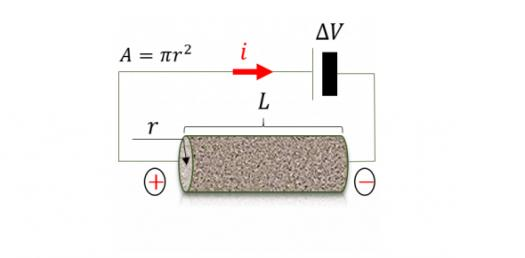# Electrical Resistivity Method MCQ Quiz

18 Questions | Attempts: 4815SettingsAre you ready for an electrical resistivity method MCQ quiz? This quiz contains some of the basic questions on the Electrical Resistivity method in Geophysics. Hope so you will enjoy the quiz. And it helps to improve your knowledge as you attempt these questions. We wish you good luck with this quiz and hope that you will give your best when you take the quiz. Let's just go for it now!

• 1.
What is the Formation Factor, given the resistivity of the matrix as 100 ohm-m and the resistivity of water as 20 ohm-m?
• A.

0.2

• B.

2.5

• C.

2.23

• D.

5

• 2.
In a 4-layer subsurface model, which combination of A-, H-, K-, and Q- type electrical resistivitysounding curves is NOT possible
• A.

HA

• B.

AK

• C.

HQ

• D.

KQ

• 3.
For three-layered earth with resistivities ρ1, ρ2, and ρ3, and corresponding thicknesses h1, h2, and h3 respectively, the quantity (h1/ρ1) + (h2/ρ2) + (h3/ρ3) stands for
• A.

Transverse Resistance

• B.

Longitudinal Conductance

• C.

Apparent Conductance

• D.

Longitudinal Resistance

• 4.
The resistivity of conducting metallic sulfides vary from:
• A.

4 to 800 ohm-m

• B.

300 to 500 ohm-m

• C.

0.00001 to 0.1 ohm-m

• D.

300 to 10000 ohm-m

• 5.
Resistivity Profiling and sounding are taken at __________ angle to the strike, respectively.
• A.

90 degree and 0 degree

• B.

0 degree and 90 degree

• C.

45 degree and 60 degree

• D.

0 degree and 45 degree

• 6.
The formation factor is related to :
• A.

Resistivity of Rock

• B.

Permeability

• C.

Porosity of Rock

• D.

The resistivity of fluid in the rock

• 7.
The limiting depth of electrical sounding is :
• A.

more than 300 m

• B.

More than 1 km

• C.

More than 5 km

• D.

More than 10 km

• 8.
The shape of a VES curve over a three-layer sequence comprising: moist soil (top), freshwater saturated coarse sand (middle), and Clay (bottom) is:
• A.

A Type

• B.

H Type

• C.

K Type

• D.

Q Type

• 9.
The electrical conduction mechanism in Sedimentary rocks is usually :
• A.

Pyroelectric

• B.

Electronic

• C.

Electrolytic

• D.

Dielectric

• 10.
Which electrode configuration has the maximum depth of investigation for homogeneous earth ????
• A.

Three electrode

• B.

Two electrode

• C.

Wenner

• D.

Schlumberger

• 11.
Which 4-electrode configuration is best suited to Archaeological Investigations ???
• A.

Pole Pole

• B.

Schlumberger

• C.

Wenner

• D.

Dipole Dipole

• 12.
The Principle of Reciprocity is applicable for both homogeneous and non-homogeneous earth.
• A.

True

• B.

False

• 13.
The resistivity of acidic igneous rocks is less than the resistivity of basic igneous rocks.
• A.

True

• B.

False

• 14.
The resistivity along a bedding plane is less than the resistivity across it.
• A.

True

• B.

False

• 15.
Which electrode configuration acquires maximum vertical resolution?
• A.

Schlumberger

• B.

Pole Dipole

• C.

Half Wenner

• D.

Wenner

• 16.
The value of coefficient of anisotropy lies between generally:
• A.

1 to 2

• B.

0 to 1

• C.

0.1 to 0.5

• D.

3 to 5

• 17.
The electrical Resistivity Method is generally used :
• A.

In the investigation of the geological structure

• B.

In engineering and groundwater investigations

• C.

For tracking dykes

• D.

For locating ore bodies

• 18.
The order of resistivity of clay is :
• A.

10 ohm-m

• B.

100 ohm-m

• C.

1000 ohm-m

• D.

5000 ohm-m

## Related TopicsBack to top
×

Wait!
Here's an interesting quiz for you.